This site uses cookies. By continuing to browse the ConceptDraw site you are agreeing to our Use of Site Cookies.
ConceptDraw Samples | Computer and Networks — Cloud Computing Diagrams

# Cloud Computing Diagrams

Samples of cloud computing diagrams are created using ConceptDraw DIAGRAM diagramming and vector drawing software enhanced with solution Cloud Computing Diagrams from ConceptDraw Solution Park.

ConceptDraw DIAGRAM provides export of vector graphic multipage documents into multiple file formats: vector graphics (SVG, EMF, EPS), bitmap graphics (PNG, JPEG, GIF, BMP, TIFF), web documents (HTML, PDF), PowerPoint presentations (PPT), Adobe Flash (SWF).

## Tutorials and Solutions:

Video Tutorials: ConceptDraw Solution Park

Solutions: Cloud Computing Diagrams for ConceptDraw DIAGRAM

## Sample 1: Anti-intrusion Sensor Architecture

Cloud Computing Diagrams sample: Anti-intrusion sensor architecture.

This example is created using ConceptDraw DIAGRAM diagramming and vector drawing software enhanced with Cloud Computing Diagrams solution from ConceptDraw Solution Park.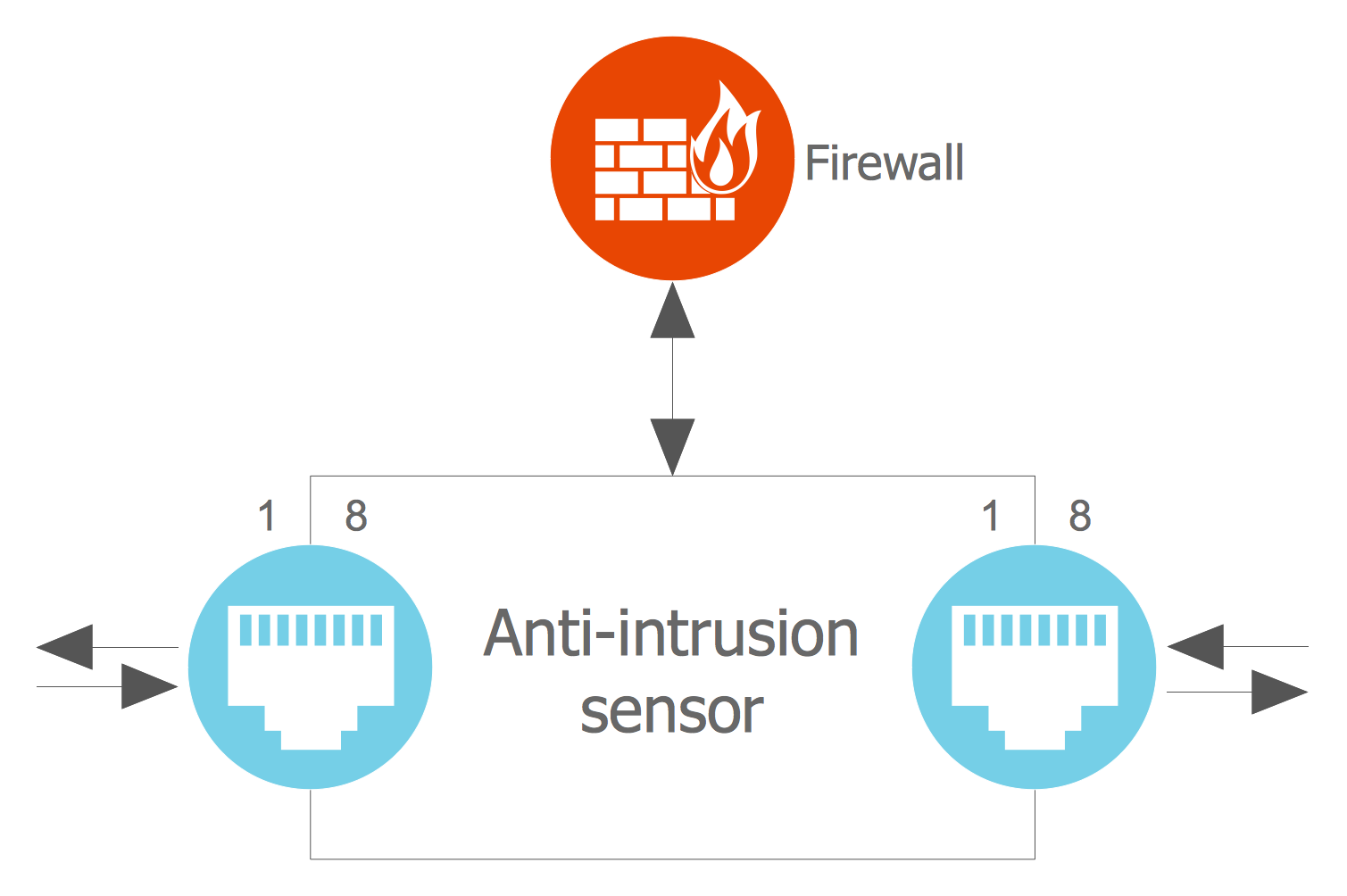## Sample 2: Cloud Applications

Cloud Computing Diagrams sample: Cloud applications.

This example is created using ConceptDraw DIAGRAM diagramming and vector drawing software enhanced with Cloud Computing Diagrams solution from ConceptDraw Solution Park.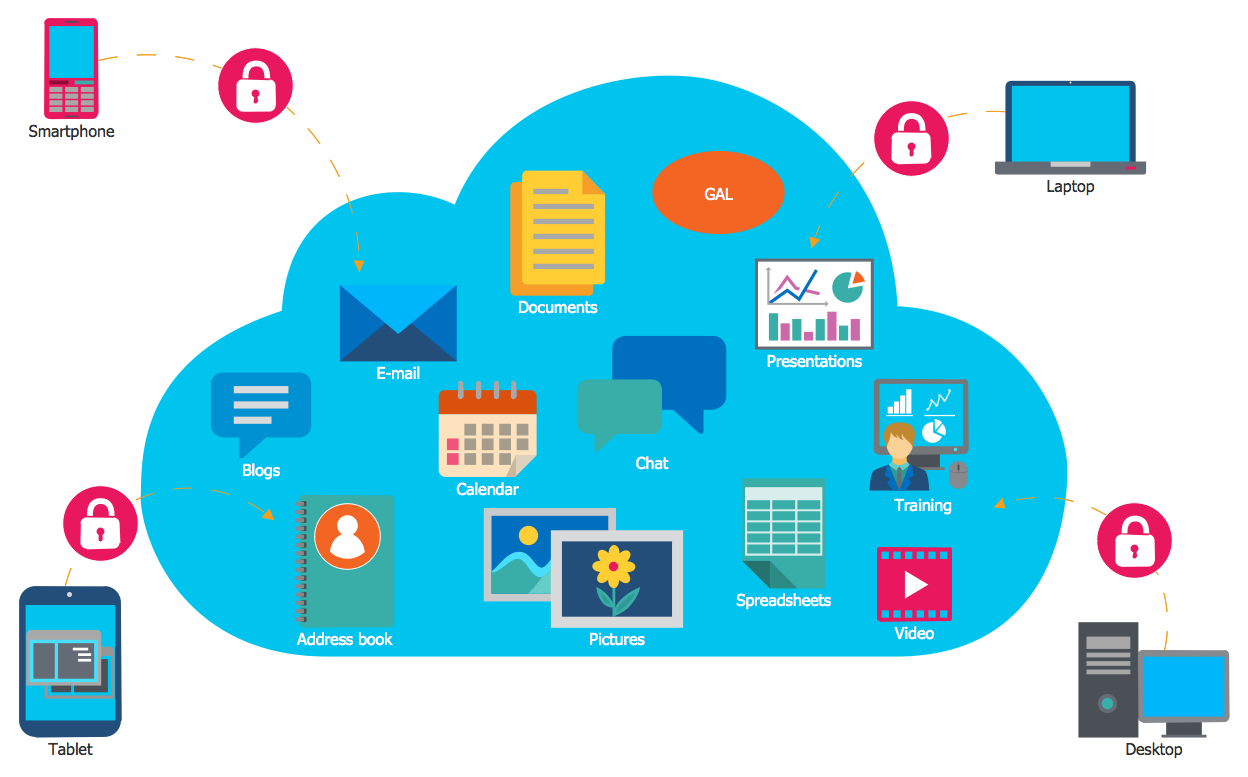## Sample 3: Cloud Computing Diagram

Cloud Computing Diagrams sample: Cloud computing diagram.

This example is created using ConceptDraw DIAGRAM diagramming and vector drawing software enhanced with Cloud Computing Diagrams solution from ConceptDraw Solution Park.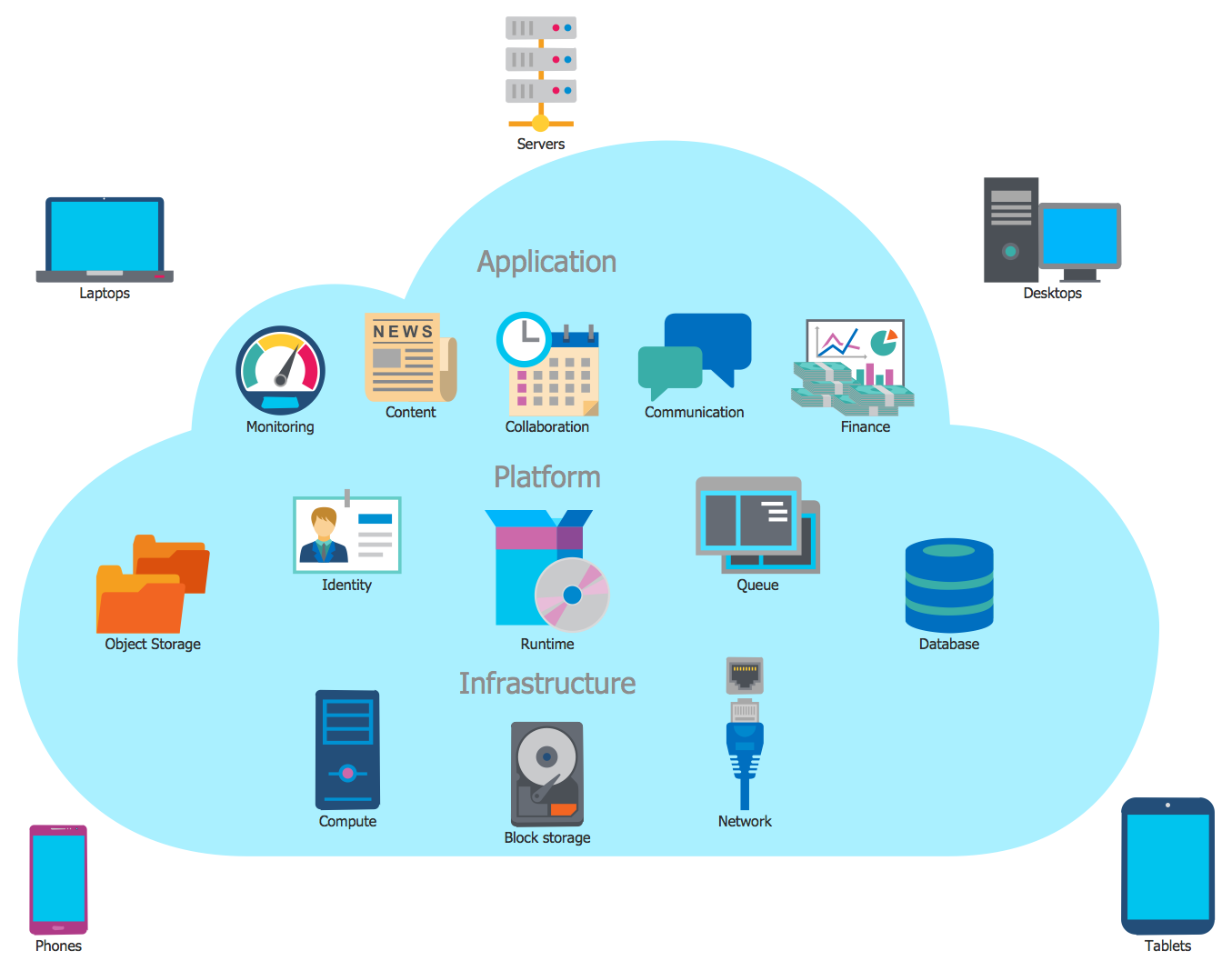## Sample 4: Cloud Computing Stack

Cloud Computing Diagrams sample: Cloud computing stack.

This example is created using ConceptDraw DIAGRAM diagramming and vector drawing software enhanced with Cloud Computing Diagrams solution from ConceptDraw Solution Park.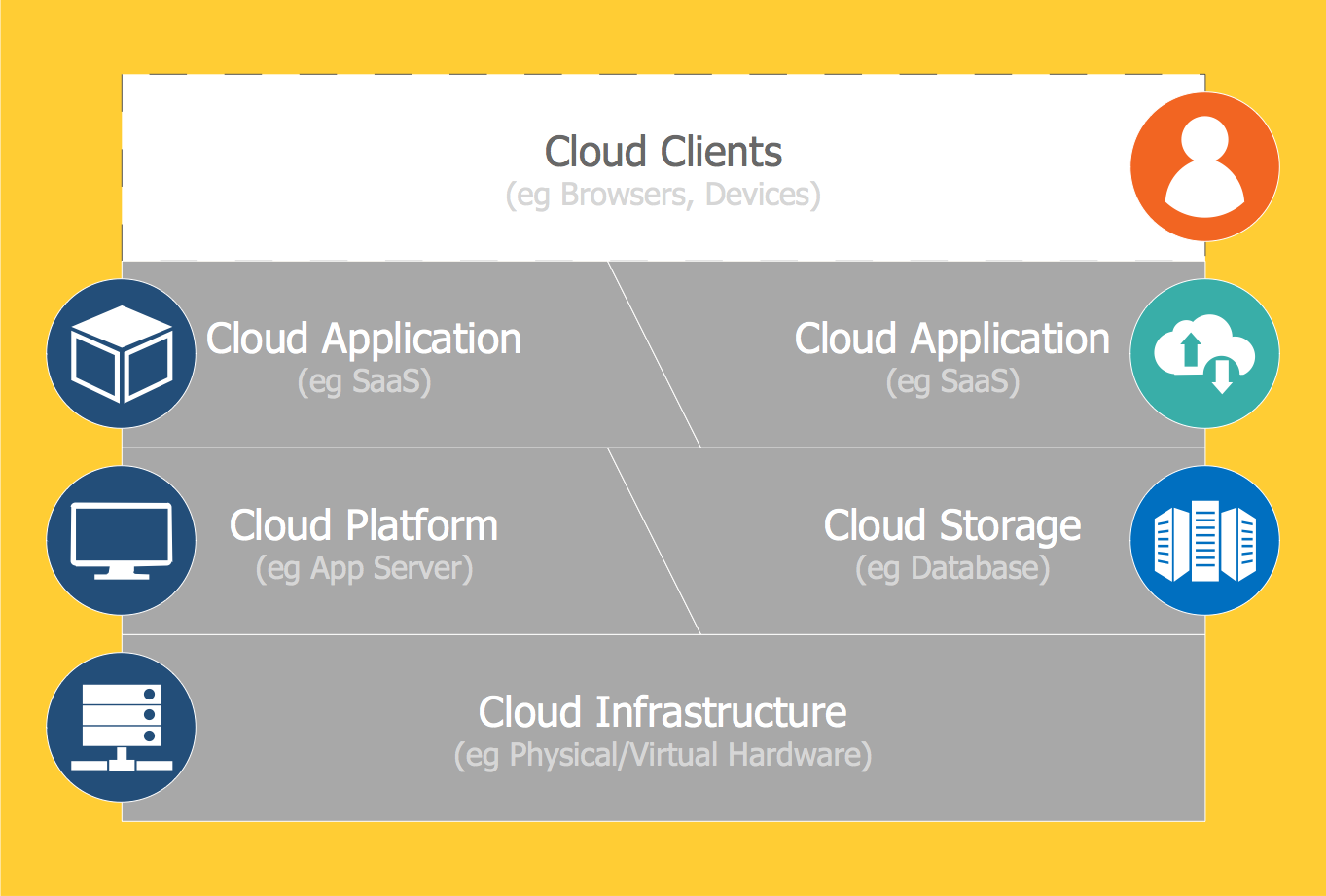## Sample 5: Cloud Computing Types

Cloud Computing Diagrams sample: Cloud computing types.

This example is created using ConceptDraw DIAGRAM diagramming and vector drawing software enhanced with Cloud Computing Diagrams solution from ConceptDraw Solution Park.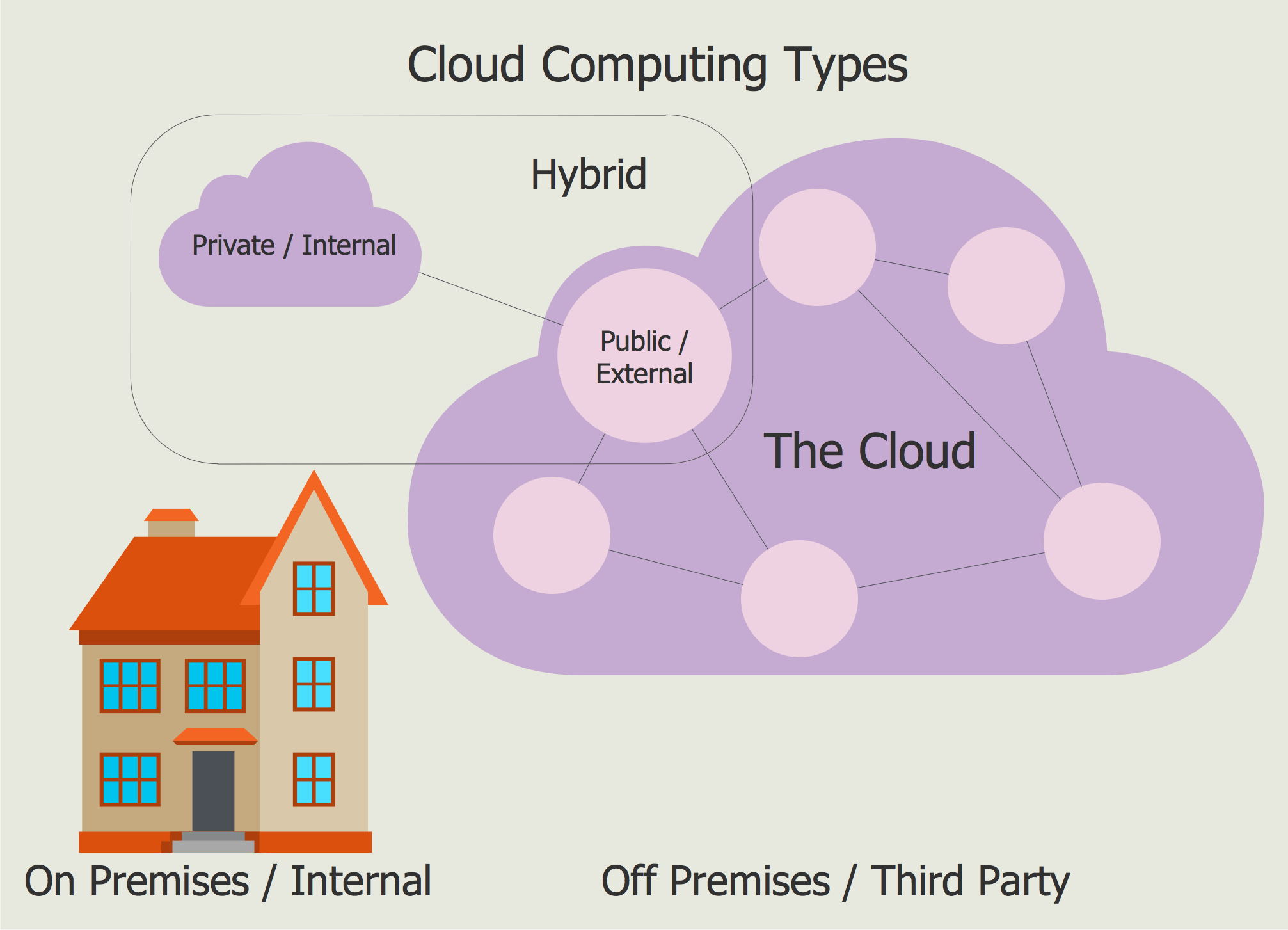## Sample 6: Cloud Computing

Cloud Computing Diagrams sample: Cloud computing.

This example is created using ConceptDraw DIAGRAM diagramming and vector drawing software enhanced with Cloud Computing Diagrams solution from ConceptDraw Solution Park.## Sample 7: Cloud Marketing Diagram

Cloud Computing Diagrams sample: Cloud marketing diagram.

This example is created using ConceptDraw DIAGRAM diagramming and vector drawing software enhanced with Cloud Computing Diagrams solution from ConceptDraw Solution Park.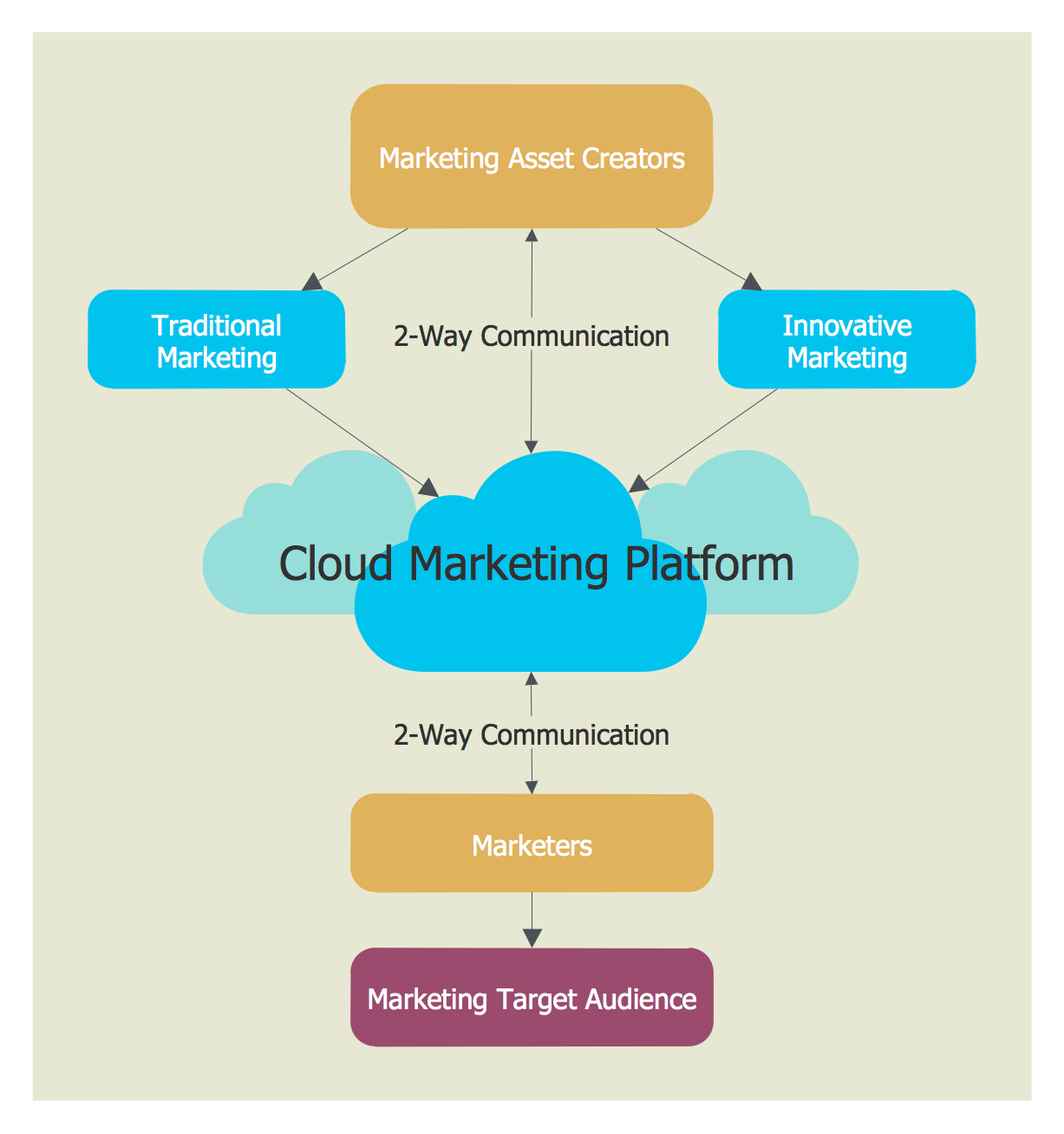## Sample 8: IBM SmartCloud Diagram

Cloud Computing Diagrams sample: IBM SmartCloud diagram.

This example is created using ConceptDraw DIAGRAM diagramming and vector drawing software enhanced with Cloud Computing Diagrams solution from ConceptDraw Solution Park.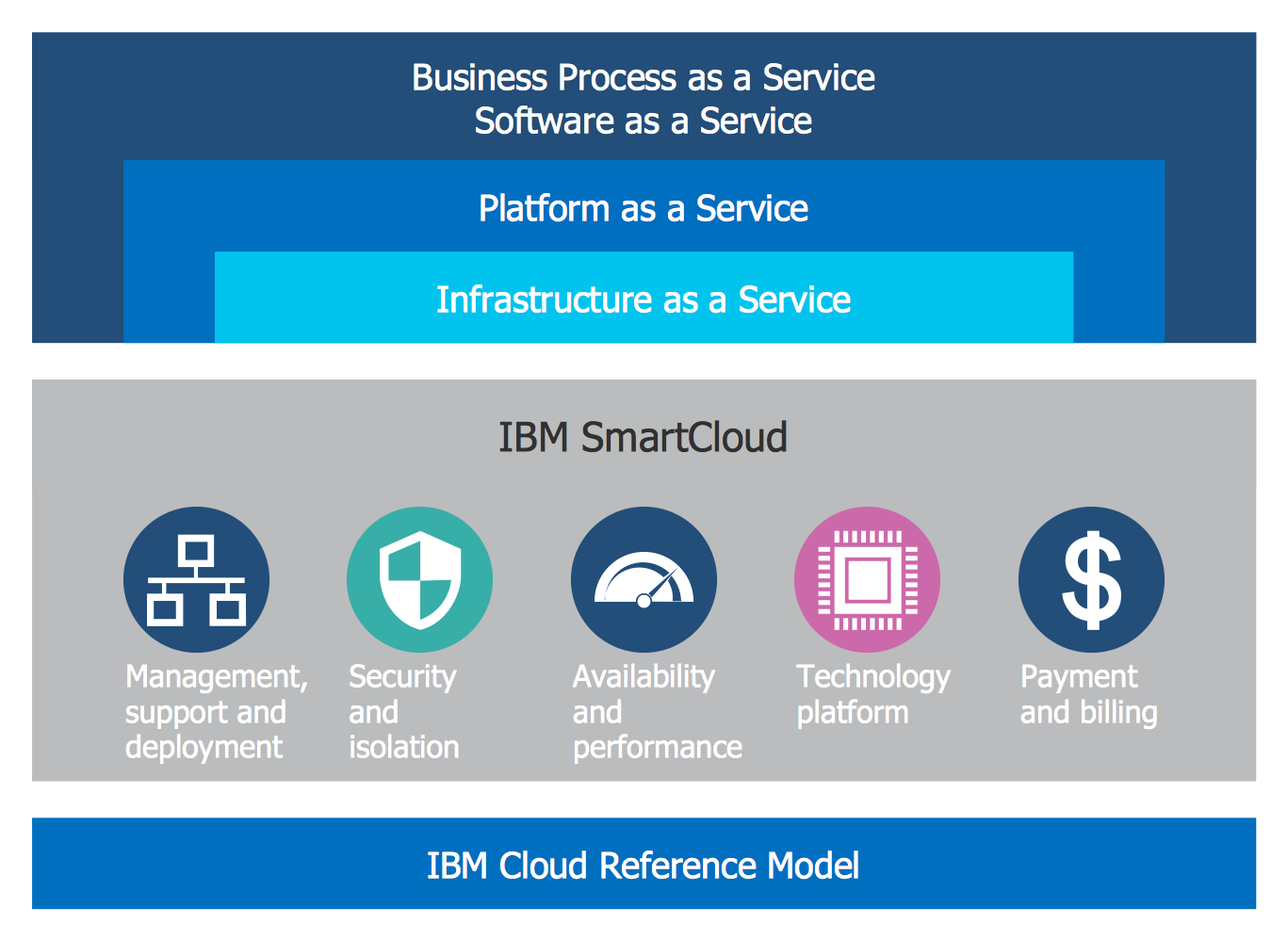## Sample 9: Principal Cloud Manufacturing

Cloud Computing Diagrams sample: Principal cloud manufacturing.

This example is created using ConceptDraw DIAGRAM diagramming and vector drawing software enhanced with Cloud Computing Diagrams solution from ConceptDraw Solution Park.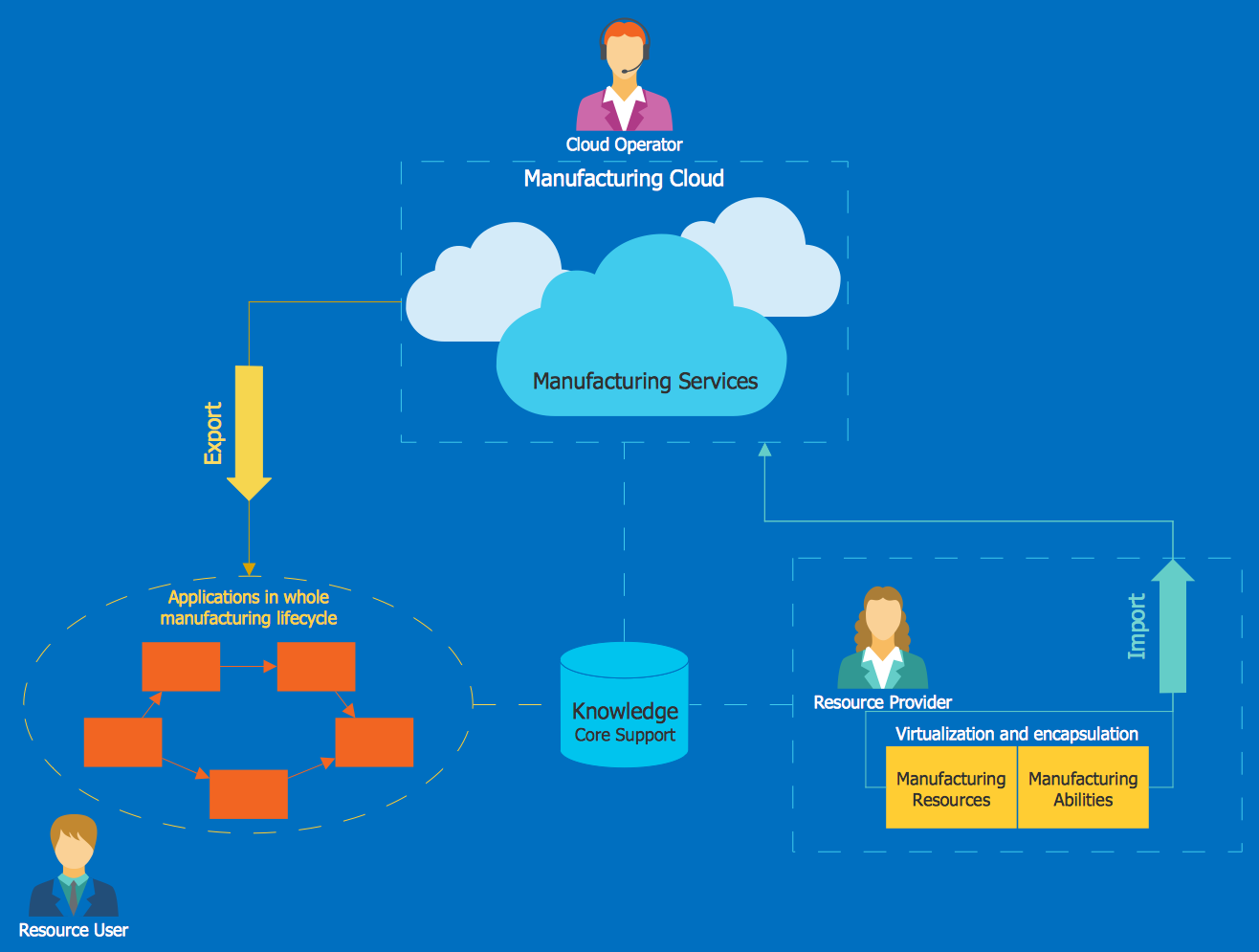## Sample 10: SmartCloud Model

Cloud Computing Diagrams sample: SmartCloud model.

This example is created using ConceptDraw DIAGRAM diagramming and vector drawing software enhanced with Cloud Computing Diagrams solution from ConceptDraw Solution Park.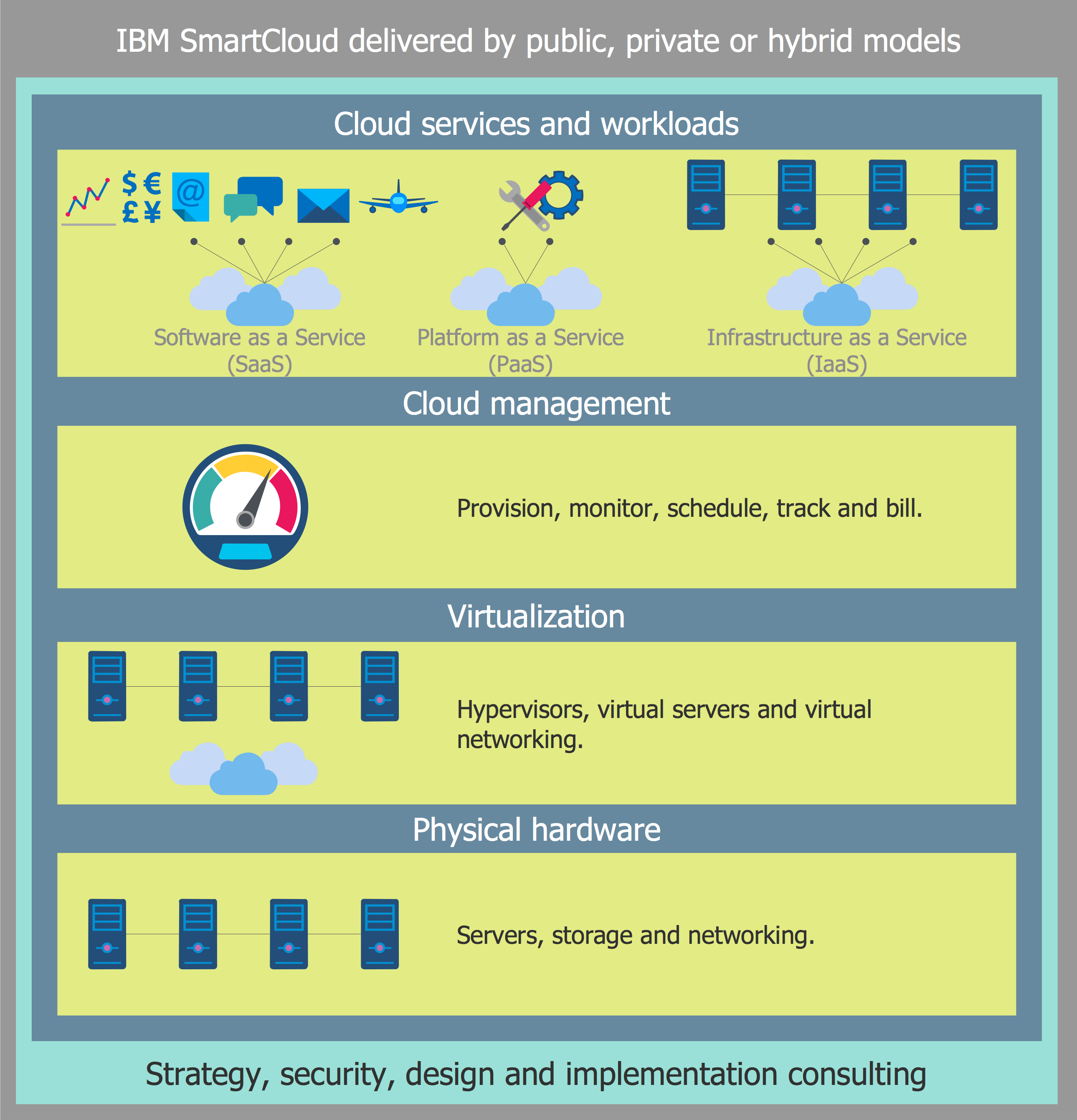All samples are copyrighted CS Odessa's.

Usage of them is covered by Creative Commons “Attribution Non-Commercial No Derivatives” License.

The text you can find at: https://creativecommons.org/licenses/by-nc-nd/3.0